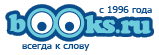Добро пожаловать! Вы можете войти или зарегистрироваться
Обратная связь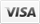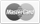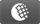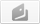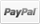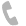+7 (495) 638-5305
+7 (812) 380-5006
Мой регион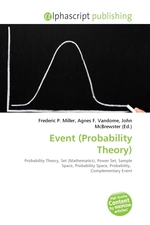# Event (Probability Theory)

бумажная книга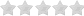(0 голосов )

• ## Действия

### Аннотация к книге "Event (Probability Theory)"

High Quality Content by WIKIPEDIA articles! In probability theory, an event is a set of outcomes (a subset of the sample space) to which a probability is assigned. Typically, when the sample space is finite, any subset of the sample space is an event (i.e. all elements of the power set of the sample space are defined as events). However, this approach does not work well in cases where the sample space is infinite, most notably when the outcome is a real number. So, when defining a probability...